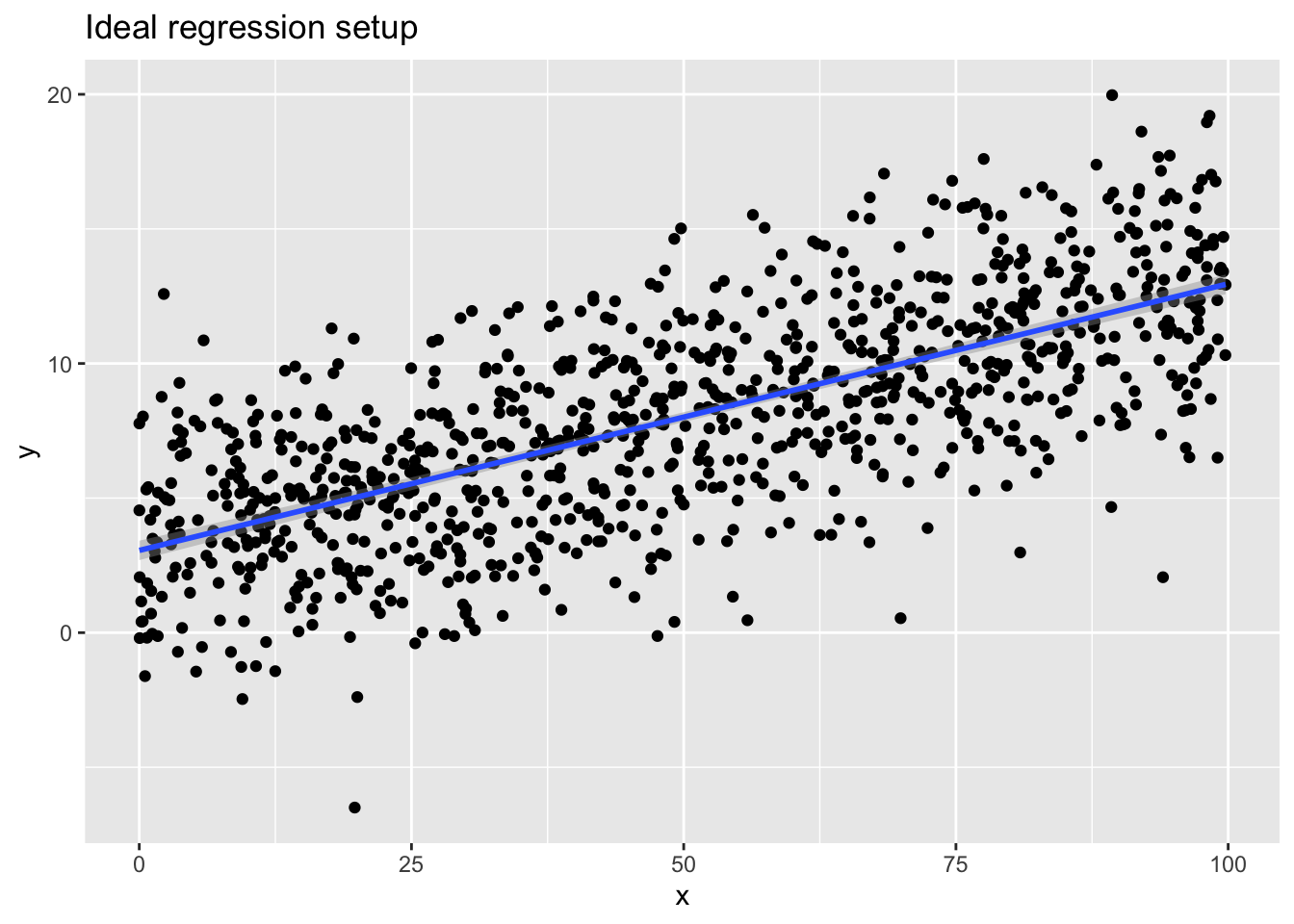library(ggplot2)
## Warning: package 'ggplot2' was built under R version 3.6.2

### Introduction

This set of supplementary notes provides further discussion of the diagnostic plots that are output in R when you run th plot() function on a linear model (lm) object.

### 1. Residual vs. Fitted plot

#### The ideal case

Let’s begin by looking at the Residual-Fitted plot coming from a linear model that is fit to data that perfectly satisfies all the of the standard assumptions of linear regression. What are those assumptions? In the ideal case, we expect the $$i$$th data point to be generated as:

$y_i = \beta_0 + \beta_1x_{1i} + \cdots + \beta_p x_{pi} + \epsilon_i$

where $$\epsilon_i$$ is an “error” term with mean 0 and some variance $$\sigma^2$$.

To create an example of this type of model, let’s generate data according to

$y_i = 3 + 0.1 x + \epsilon_i,$

for $$i = 1, 2, \ldots, 1000$$, where the $$\epsilon_i$$ are independent Normal$$(0,sd = 3)$$ variables (with standard deviation 3).

Here’s code to generate this data and then regress y on x.

n <- 1000      # sample size
x <- runif(n, min = 0, max = 100)
y.good <- 3 + 0.1 * x + rnorm(n, sd = 3)

# Scatterplot of the data with regression line overlaid
qplot(x, y.good, ylab = "y", main = "Ideal regression setup") + stat_smooth(method = "lm")
## geom_smooth() using formula 'y ~ x'# Run regression and display residual-fitted plot
lm.good <- lm(y.good ~ x)
plot(lm.good, which = 1)